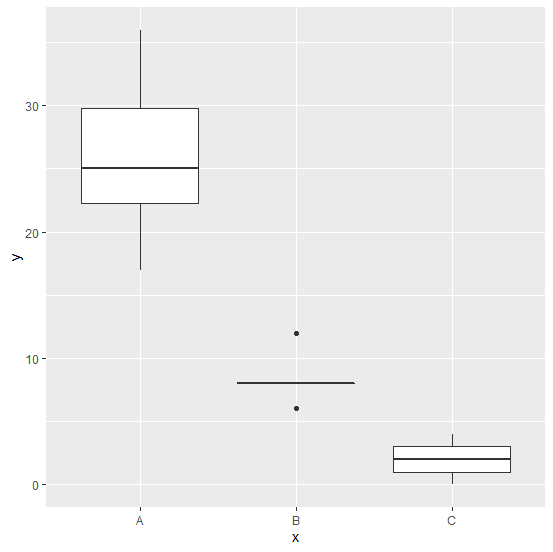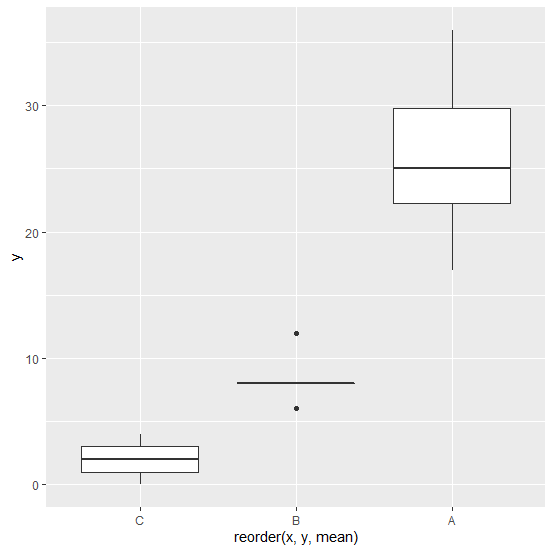# How to change the order of boxplot by means using ggplot2 in R?

R ProgrammingServer Side ProgrammingProgramming

To change the order of boxplot by means using ggplot2, we can use reorder function inside aes of ggplot. For example, if we have a data frame called df that contains two columns say x (categorical) and y(count) then the boxplot ordered by means can be created by using the command ggplot(df,aes(x=reorder(x,y,mean),y))+geom_boxplot()

## Example

Consider the below data frame −

Live Demo

> x<-c(rep(c("A","B","C"),times=c(10,5,5)))
> y<-c(rpois(10,25),rpois(5,10),rpois(5,2))
> df<-data.frame(x,y)
> df

## Output

   x  y
1  A 22
2  A 17
3  A 20
4  A 36
5  A 34
6  A 25
7  A 25
8  A 30
9  A 23
10 A 29
11 B  8
12 B  8
13 B  6
14 B  8
15 B 12
16 C  0
17 C  4
18 C  3
19 C  2
20 C  1

> library(ggplot2)
> ggplot(df,aes(x,y))+geom_boxplot()

## OutputCreating boxplot for categories in x ordered by mean of y −

> ggplot(df,aes(x=reorder(x,y,mean),y))+geom_boxplot()

## Output